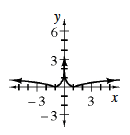### Home > A2C > Chapter 6 > Lesson 6.2.5 > Problem6-118

6-118.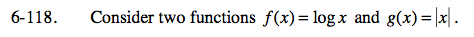Use the eTool below to view the graph of the equations and solve the parts of the problem.
Click the link at right for the full version of the eTool: A2C 6-118 HW eTool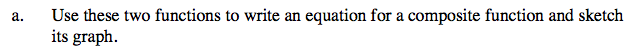A composition of two functions is when the output of the first function becomes the input of the second.

g(f(x)) = |log(x)| or
f(g(x)) = log|x|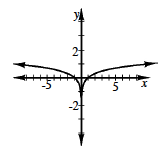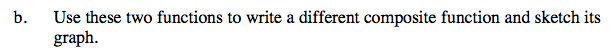See part (a).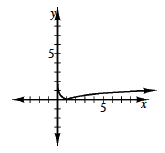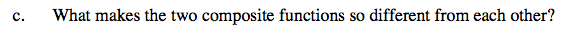Remember that the order of composition matters.

When using the absolute value first, you can use both positive and negative numbers for x, so the domain is different from the domain for the log function.When using the log first and then taking the absolute value, only positive values can be used for x. If the result of the log is negative, the absolute value makes it positive, so the range is only positive numbers.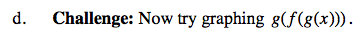Think this through; you can do it!